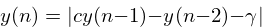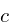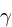ChaosNoise

# ChaosNoise

## Overview

The chaosnoise algorithm is noise generator that uses a chaos function to produce sound.

At it's core, chaosnoise is essentially an unstable 2-pole filter with a nonlinearity. In other words, it makes noise doing things DSP engineers work very hard to avoid under normal circumstances.

The underlying chaos function is largely based on the one found in the Crackle Ugen found in SuperCollider. In this implementation, however, there is an added rate parameter similar to the one found in bitnoise. This is used to change the tone quality of the algorithm.

## Tangled Files

This tangles to chaosnoise.c and chaosnoise.h. Defining SK_CHAOSNOISE_PRIV will expose the core struct in the header file.

<<chaosnoise.c>>=
#include <math.h>
#define SK_CHAOSNOISE_PRIV
#include "chaosnoise.h"
<<macros>>
<<funcs>>

<<chaosnoise.h>>=
#ifndef SK_CHAOSNOISE_H
#define SK_CHAOSNOISE_H

#ifndef SKFLT
#define SKFLT float
#endif

<<typedefs>>
<<funcdefs>>

#ifdef SK_CHAOSNOISE_PRIV
<<structs>>
#endif

#endif

## Struct

Data for ChaosNoise is contained in a struct called sk_chaosnoise.

<<typedefs>>=
typedef struct sk_chaosnoise sk_chaosnoise;

<<structs>>=
struct sk_chaosnoise {
<<sk_chaosnoise>>
};

## Filter Memory

chaosnoise is technically a 2-pole filter, requiring memory for 2 samples of signal.

<<sk_chaosnoise>>=
SKFLT y;

<<init>>=
cn->y = init;
cn->y = 0;

## Sample and Hold Constants and Parameters

The signal is clocked with a sample and hold generator. This sample and hold will use a fixed-point phasor to keep track of time, similar to the ones found in bitnoise and rline. More details on how this works can be found in these pages.

A 32-bit integer called phs keeps track of the phase position.

<<sk_chaosnoise>>=
long phs;

<<init>>=
cn->phs = 0;

The constant SK_CHAOSNOISE_PHSMAX defines the maximum length (and resolution) of the phasor, set to be 0x1000000.

<<macros>>=
#define SK_CHAOSNOISE_PHSMAX 0x1000000L

The constant SK_CHAOSNOISE_PHSMSK defines the masking value of the phasor, set to be 0x0FFFFFF.

<<macros>>=
#define SK_CHAOSNOISE_PHSMSK 0x0FFFFFFL

The floating point value maxlens is SK_CHAOSNOISE_PHSMAX, converted to seconds. It is used to calculate the increment amount for the phase position. When a rate value (in units of Hz, or cycles/second) get multiplied by this value, the result is a value in units of phase cycles.

<<sk_chaosnoise>>=
SKFLT maxlens;

<<init>>=
cn->maxlens = SK_CHAOSNOISE_PHSMAX / (SKFLT) sr;

## Parameters

### Chaos

The chaos parameter is variable that controls the chaos level. It is typically a value between 1 and 2.

It can be set with sk_chaosnoise_chaos.

<<funcdefs>>=
void sk_chaosnoise_chaos(sk_chaosnoise *cn, SKFLT chaos);

<<funcs>>=
void sk_chaosnoise_chaos(sk_chaosnoise *cn, SKFLT chaos)
{
cn->chaos = chaos;
}

<<sk_chaosnoise>>=
SKFLT chaos;

<<init>>=
sk_chaosnoise_chaos(cn, 1.5);

### Rate

The rate value controls the rate of computation. This is a value measure in Hertz.

It can be set with sk_chaosnoise_rate.

<<funcdefs>>=
void sk_chaosnoise_rate(sk_chaosnoise *cn, SKFLT rate);

<<funcs>>=
void sk_chaosnoise_rate(sk_chaosnoise *cn, SKFLT rate)
{
cn->rate = rate;
}

<<sk_chaosnoise>>=
SKFLT rate;

<<init>>=
sk_chaosnoise_rate(cn, 8000);

## Initialization

Initialized with sk_chaosnoise_init. Sampling rate and an initial value must be supplied as arguments. The initial value is a floating point value that expects to be in range 0-1.

<<funcdefs>>=
void sk_chaosnoise_init(sk_chaosnoise *cn, int sr, SKFLT init);

<<funcs>>=
void sk_chaosnoise_init(sk_chaosnoise *cn, int sr, SKFLT init)
{
<<init>>
}

## Computation

A single sample of audio is computed with sk_chaosnoise_tick.

<<funcdefs>>=
SKFLT sk_chaosnoise_tick(sk_chaosnoise *cn);

<<funcs>>=
SKFLT sk_chaosnoise_tick(sk_chaosnoise *cn)
{
SKFLT out;
out = 0;

<<update_phasor>>
<<compute_noise>>
<<copy_to_out>>

return out;
}

First, the phasor value is updated by an increment value, computed with rate*maxlens.

<<update_phasor>>=
cn->phs += floor(cn->rate * cn->maxlens);

If the phasor reaches (or goes above) the end, a new sample gets computed.

This uses the following difference equation:Whereis the chaos value, andis a nonlinearity set to be 0.05.

After the output value is computed, the coefficients are updated.

<<compute_noise>>=
if (cn->phs >= SK_CHAOSNOISE_PHSMAX) {
SKFLT y;

cn->phs &= SK_CHAOSNOISE_PHSMSK;
y = fabs(cn->chaos * cn->y - cn->y - 0.05);
cn->y = cn->y;
cn->y = y;
}

The output is always set to be the filter memory variable y.

<<copy_to_out>>=
out = cn->y;